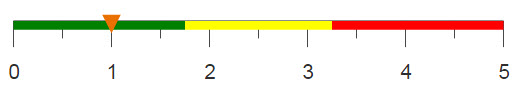# Transposing a table

In order to transpose a table using SQL, multiple operations are required. Using 1010data, you can transpose a table with one basic operation.

## Difficulty## Objective

You have determined the top 5 selling products based on the sum sales for transactions occurring in 2015. Now that you have a column containing the top five SKUs, you want to transpose the table so that each SKU is in it's own column.

## SQL solution

```SET @row_num=0;
SELECT MAX(CASE WHEN rank=1 THEN sku END) AS first,
MAX(CASE WHEN rank=2 THEN sku END) AS second,
MAX(CASE WHEN rank=3 THEN sku END) AS third,
MAX(CASE WHEN rank=4 THEN sku END) AS fourth,
MAX(CASE WHEN rank=5 THEN sku END) AS fifth
FROM (
SELECT sku,
@row_num := @row_num + 1 AS rank
FROM (
SELECT sku,
sum(xsales) AS sum_sales
FROM sales_detail
WHERE YEAR(trans_date)=2015
GROUP BY sku
ORDER BY sum_sales DESC
LIMIT 5
) sales_by_sku
) top_ranked;```

In order to transpose a table in SQL, you have to create each new column in your SELECT statement and specify which values you want in each. In this example, it is not too intensive to create five additional columns containing only one type of information, however this becomes more difficult when transposing a larger, more complex table. Additionally, in order to transpose only the sku column, you need to create a column containing the row index, which is rank in this example.

## 1010data Macro Language solution

```<base table="pub.doc.retail.altseg.sales_detail_sku"/>
<sel value="year(trans_date)=2015"/>
<willbe name="sales_per_sku" value="g_sum(sku;;xsales)" format="dec:2"/>
<sel value="g_first1(sku;;)"/>
<sort col="sales_per_sku" dir="down"/>
<sel value="i_()<=5"/>
<colord cols="sku"/>
<willbe name="names"
value="case(i_();1;'first';2;'second';3;'third';4;'fourth';'fifth')"/>
<transpose names="names"/>```

With 1010data, one operation transposes your table- <transpose/>. This operation offers many attributes that give you the ability to customize your transposed table. In this analogue, the names attribute is used in order to name each column in the transposed table. Any column containing data formatted as text, can be used with this attribute. Additionally, there are attributes that allow you to label the columns of your new table , labels, and attributes that allow you to have data of different types transposed into one column, promote, among others.

## Further reading

If you would like to learn more about the functions and operations discussed in this recipe, click on the links below:

<transpose>